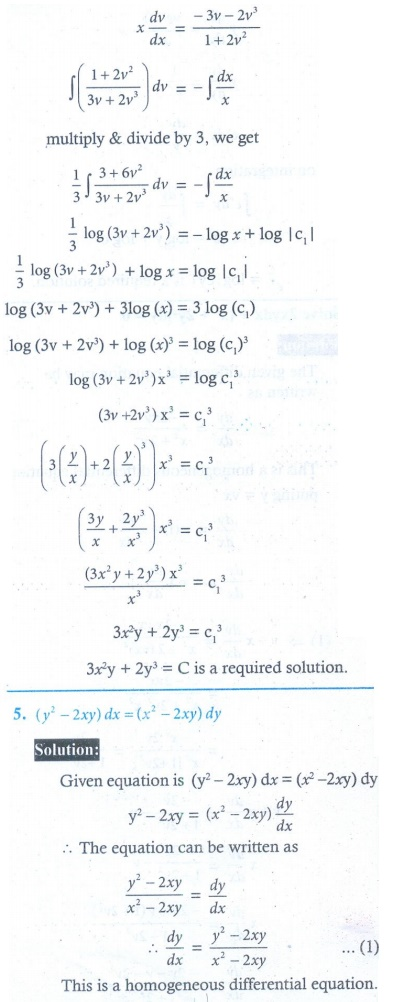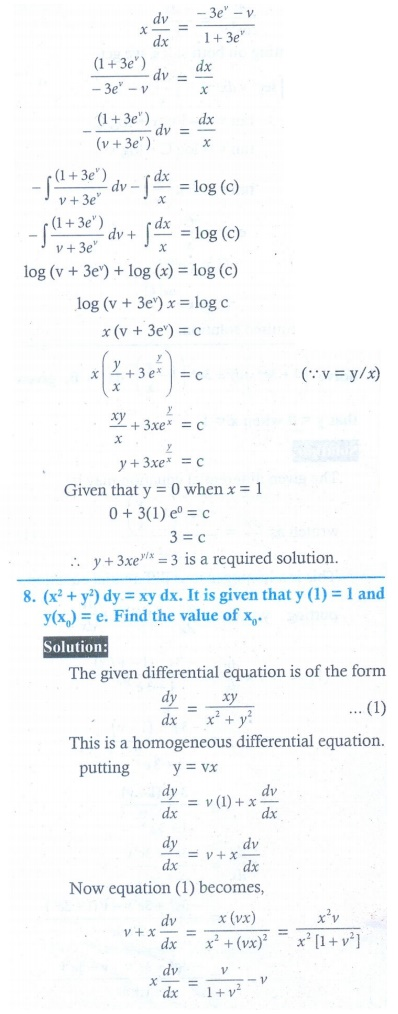Home | | Maths 12th Std | Exercise 10.6: Homogeneous Form or Homogeneous Differential Equation

# Exercise 10.6: Homogeneous Form or Homogeneous Differential Equation

Maths Book back answers and solution for Exercise questions - Mathematics : Ordinary Differential Equations: Solution of First Order and First Degree Differential Equations: Homogeneous Form or Homogeneous Differential Equation - Exercise Problem Questions with Answer, Solution

EXERCISE 10.6

Solve the following differential equations:8. ( x 2 + y2 )dy = xy dx . It is given that y (1) = 1and y ( x0 ) = e . Find the value of x0 .